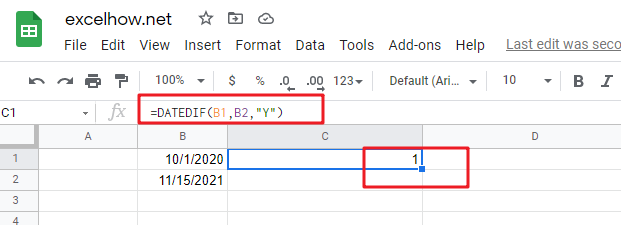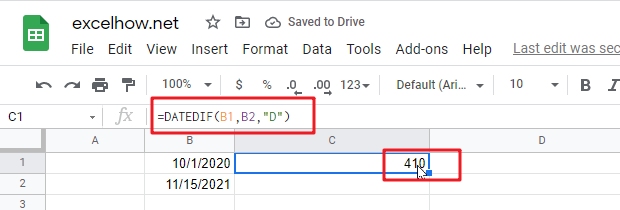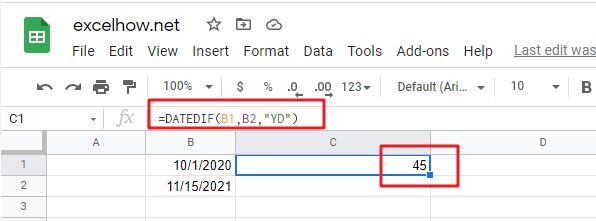This post will guide you how to use Google Sheets DATEDIF function with syntax and examples.

## Description

The Google Sheets DATEDIF returns the number of days, months, or years between tow dates.

The DATEDIF function can be used to calculate the date between a start date and an end date in year, month or days. The purpose of this function is to get days, months or years between two given dates and its returned values is a number.

The DATEDIF function is a build-in function in Google Sheets and it is categorized as a Date function.

## Syntax

The syntax of the DATEDIF function is as below:

=DATEDIF (start_date,end_date,unit)

Where the DATEDIF function arguments are:

• Start_date – The start date to consider in the calculation. It can be a cell reference that containing a DATE value, or a number.
• End_date – the end date to consider in the calculation. It must be a cell reference that containing a DATE value or a number.
• Unit the type of information that you want to return.

Note:

• The index_num value must be a number between 1 and 29. The DATEDIF function can accpet Unit values are `"Y"`,`"M"`,`"D" `,`"MD"`,`"YM"`,`"YD"`.
• “Y”– The number of complete years
• “M”– The number of complete months
• “D”– The number of days
• “MD”– The difference between the days in start_date and end_date.
• “YM”– The difference between the months in start_date and end_date
• “YD”– The difference between the days (the years of dates are ignored)
• If Start_date is greater than the end_date, the DATEDIF will return a #NUM! error message. You can trap this error with the IFERROR function in combination with the DATEDIF function.

## Google Sheets DATEDIF Function Examples

The below examples will show you how to use google sheets DATEDIF Function to return the difference between two dates.

#1 =DATEDIF(B1,B2,”Y”)Note: the above google sheets formula will return the number of complete year as “1” between start_date (10/1/2020) and end_date(11/15/2021).

#2 =DATEDIF(B1,B2,”D”)Note: the above formula will return the number of days (410) between 10/1/2020 and 11/15/2021.

#3 =DATEDIF(B1,B2,”YD”)Note: the above formula will return 45 days between 10/1 and 11/15, ignoring the years of dates.